# Gyromagnetic Ratio

Gyromagnetic Ratio is defined by: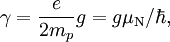$\gamma = \frac{e}{2m_p}g = g \mu_\mathrm{N}/\hbar,$

where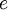$\displaystyle e$ is the magnitude of electron charge,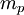$\displaystyle m_p$ is the proton mass,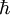$\displaystyle \hbar$ is reduced Planck constant,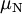$\displaystyle \mu_\mathrm{N}$ is the nuclear magneton, and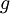$\displaystyle g$ is the g-factor of the nucleon or nucleus in question.

Approximate values for some common nuclei are given in the Table below.<ref>Template:Cite book</ref>

species γ / 2π (MHz/T) 
free electron 2.80249536 x 104 
1H 42.576
2D 6.53593
3He -32.434
7Li 16.546
13C 10.705
14N 3.0766
15N -4.3156
17O -5.7716
19F 40.0593
23Na 11.262
31P 17.235
75As 7.2919
129Xe -11.777

For information on individual chemical elements please use NMR and EPR Periodic Table

To find NMR frequency of your favorite nucleus at a given magnetic field, see Alex Jerschow's on-line Interactive NMR Frequency Map

## References

1. values are divided by 2*pi, those in nist tables are not [nistval]
2. gyromagnetic ratio of free electron

[niste]